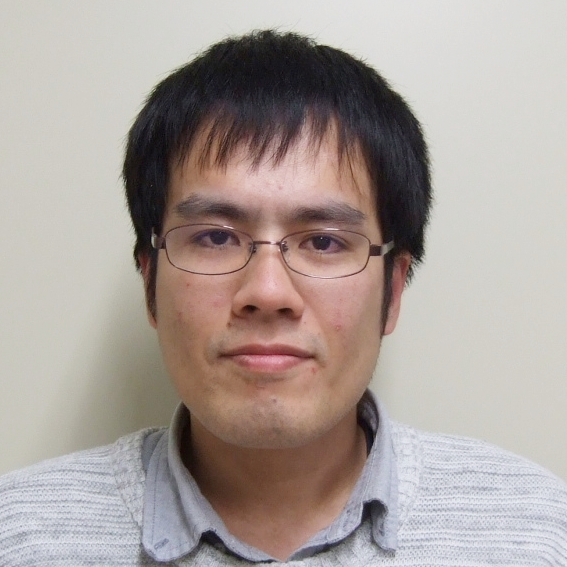## HASEBE TakahiroAssociate Professor

### Probability theory for non-commuting variables

Department of Mathematics, MathematicsTheme Noncommutative probability theory Field Pprobability theory, funcitonal analysis, combinatorics Keyword Free probability, infinitely divisible distributions, Levy processes, cumulants

#### Introduction of Research

The meaning of a word changes once the order of the alphabets constituting the word is changed. The theory of non-commutative probability is based on regarding such non-commuting elements as "random variables". How to define "independence" is a central subject to be understood. The applications of this research field include random matrices, quantum information, representation theory of groups and graph theory.

#### Representative Achievements

T. Hasebe and H. Saigo, The monotone cumulants, Ann. Inst. Henri Poincare Probab. Stat. 47, No. 4 (2011), 1160-1170.
T. Hasebe and S. Thorbjørnsen, Unimodality of the freely selfdecomposable probability laws, J. Theoret. Probab. 29 (2016), Issue 3, 922-940.
O. Arizmendi and T. Hasebe, Limit theorems for free Levy processes, Electron. J. Probab. 23, no. 101 (2018), 36 pp.#### Belongs